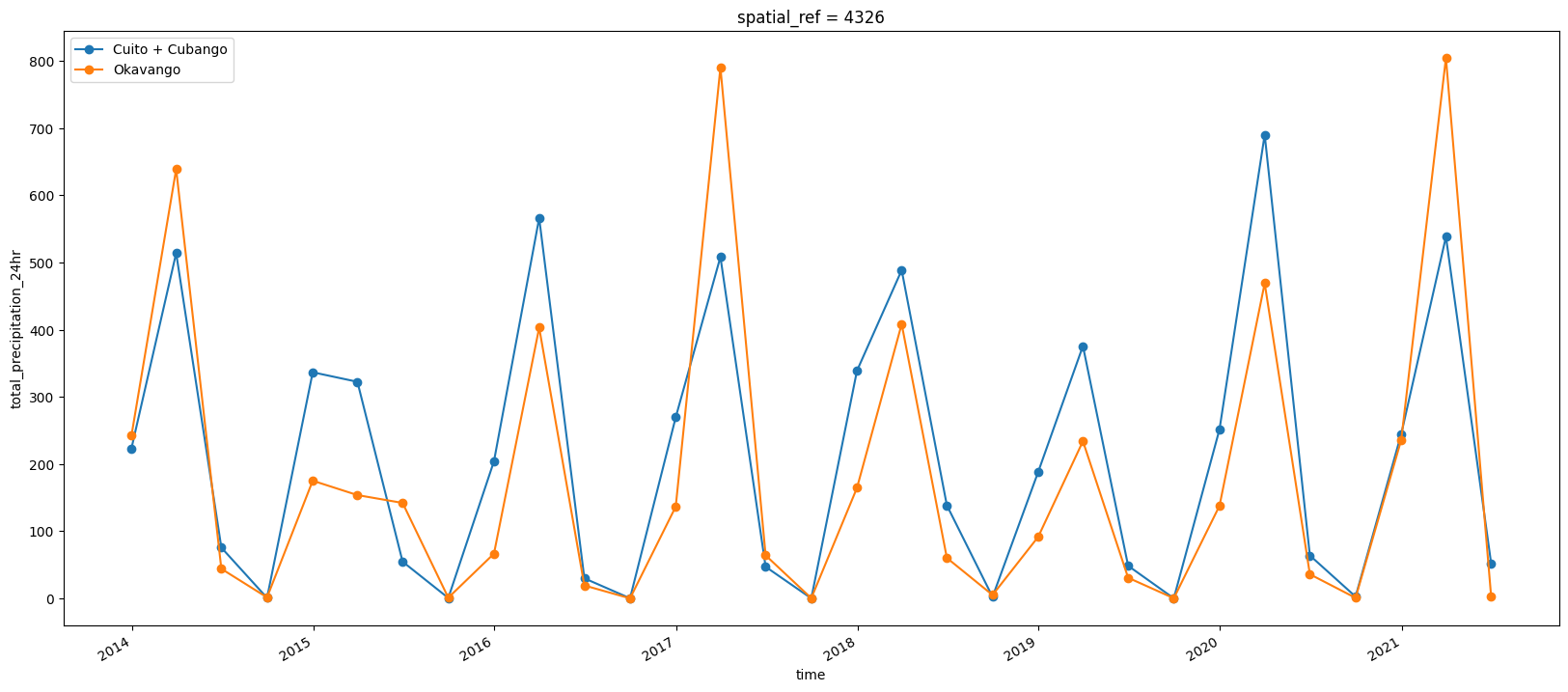# Extracting historical climate (rainfall) data over selected basins¶

• Products used: ERA5

## Getting started¶

To run this analysis, run all the cells in the notebook, starting with the “Load packages” cell.

Import Python packages that are used for the analysis.

:

%matplotlib inline

# Force GeoPandas to use Shapely instead of PyGEOS
# In a future release, GeoPandas will switch to using Shapely by default.
import os
os.environ['USE_PYGEOS'] = '0'

import datacube
import matplotlib.pyplot as plt
import numpy as np
import pandas as pd
import geopandas as gpd

from deafrica_tools.spatial import xr_rasterize


## Analysis Parameters¶

:

# Original shapefile from https://data.apps.fao.org/map/catalog/srv/api/records/57bb1c95-2f00-4def-886f
vector_file = 'data/OB_FWR_Hydrography_Okavango_Subasins_polygon.geojson'

# define time period of interest
time_range = '2013-12', '2021-05-31'


## Define areas of interest in the Okavango Basin¶

:

# load basin polygons
# Original shapefile from https://data.apps.fao.org/map/catalog/srv/api/records/57bb1c95-2f00-4def-886f-caee3d756da9

# upstream include Cuito and Cubango subbasins
upstream = basin[basin.Subbasin.isin(['Cuito', 'Cubango'])]

# delta is part of the Okavango subbasin
delta = basin[basin.Subbasin.isin(['Okavango'])]

print(upstream)

   CLASS_ID  Longitude   Latitude Subbasin      Sph_Area      Sph_Len  Parts  \
0         0  19.003252 -15.179938    Cuito  5.902293e+10  2285720.103    1.0
1         0  17.870032 -15.740153  Cubango  1.078813e+11  3071839.684    1.0

Vertices                                           geometry
0    9326.0  POLYGON ((17.85205 -13.53846, 17.85205 -13.536...
1   11314.0  POLYGON ((15.99370 -13.93323, 15.99370 -13.931...


## Retrieve historical rainfall data over all areas of interest¶

:

# get historical rainfall for upstream and delta
bounds = pd.concat([upstream, delta]).total_bounds
lat = bounds, bounds
lon = bounds, bounds

:

# download ERA5 rainfall and aggregate to monthly
var = 'precipitation_amount_1hour_Accumulation'
precip_1W = load_era5(var, lat, lon, time_range, reduce_func=np.sum, resample='1W').compute()

#resample to 3 month seasons
precip = precip_1W.resample(time='3M').sum()

# fix inconsistency in axis names
precip = precip.rename({'lat':'latitude', 'lon':'longitude'})
precip_1W = precip_1W.rename({'lat':'latitude', 'lon':'longitude'})


## Create area mask for upstream and okavango regions¶

:

upstream_raster = xr_rasterize(upstream, precip)
delta_raster = xr_rasterize(delta, precip)


## Calculate the total rainfall over each area¶

:

upstream_rainfall = precip[var].where(upstream_raster).sum(['latitude','longitude'])
okavango_rainfall = precip[var].where(delta_raster).sum(['latitude','longitude'])

upstream_rainfall_1W = precip_1W[var].where(upstream_raster).sum(['latitude','longitude'])

:

upstream_rainfall_1W = precip_1W[var].where(upstream_raster).sum(['latitude','longitude'])


## Export results as csv¶

:

upstream_rainfall.to_dataframe().drop('spatial_ref',axis=1).rename({'precipitation_amount_1hour_Accumulation':'cumulative 3-month rainfall (mm)'},axis=1).to_csv(f'results/upstream_rainfall_{time_range}_to_{time_range}.csv')
okavango_rainfall.to_dataframe().drop('spatial_ref',axis=1).rename({'precipitation_amount_1hour_Accumulation':'cumulative 3-month rainfall (mm)'},axis=1).to_csv(f'results/okavango_rainfall_{time_range}_to_{time_range}.csv')

upstream_rainfall_1W.to_dataframe().drop('spatial_ref',axis=1).rename({'precipitation_amount_1hour_Accumulation':'cumulative 1-week rainfall (mm)'},axis=1).to_csv(f'results/upstream_rainfall_1W_{time_range}_to_{time_range}.csv')


## Visualize historical rainfall and compare to water extent changes¶

:

upstream_rainfall.plot(label='Cuito + Cubango', marker='o', figsize=(20,8));
okavango_rainfall.plot(label='Okavango', marker='o');
plt.legend();Contact: If you need assistance, please post a question on the Open Data Cube Slack channel or on the GIS Stack Exchange using the open-data-cube tag (you can view previously asked questions here). If you would like to report an issue with this notebook, you can file one on Github.

Compatible datacube version:

:

print(datacube.__version__)

1.8.15


Last Tested:

:

from datetime import datetime
datetime.today().strftime('%Y-%m-%d')

:

'2023-08-21'